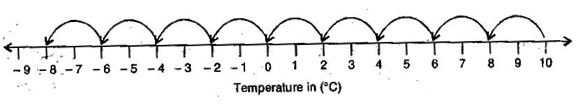### Integers - Solutions 4

CBSE Class –VII Mathematics
NCERT Solutions
Chapter 1 Integers
(Ex. 1.4)

Question 1. Evaluate each of the following:
(a) $\left(-30\right)÷10$
(b) $50÷\left(-5\right)$
(c) $\left(-36\right)÷\left(-9\right)$
(d) $\left(-49\right)÷49$
(e) $13÷\left[\left(-2\right)+1\right]$
(f) $0÷\left(-12\right)$
(g) $\left(-31\right)÷\left[\left(-30\right)+\left(-1\right)\right]$
(h) $\left[\left(-36\right)÷12\right]÷3$
(i) $\left[\left(-6\right)+5\right]÷\left[\left(-2\right)+1\right]$
Answer: (a) $\left(-30\right)÷10$ = $\left(-30\right)×\frac{1}{10}=\frac{-30×1}{10}=-3$
(b) $50÷\left(-5\right)$ = $50×\left(\frac{-1}{5}\right)=\frac{50×\left(-1\right)}{5}=-10$
(c) $\left(-36\right)÷\left(-9\right)$ = $\left(-36\right)×\left(\frac{-1}{9}\right)=\frac{\left(-36\right)×\left(-1\right)}{9}=\frac{36}{9}=4$
(d) $\left(-49\right)÷49$ = $\left(-49\right)×\frac{1}{49}=\frac{-49}{49}=-1$
(e) $13÷\left[\left(-2\right)+1\right]$ = $13÷\left(-1\right)=13×\left(\frac{-1}{1}\right)=-13$
(f) $0÷\left(-12\right)$ = $0×\left(\frac{-1}{12}\right)=\frac{0}{12}=0$
(g) $\left(-31\right)÷\left[\left(-30\right)+\left(-1\right)\right]$ = $\left(-31\right)÷\left(-30-1\right)=\left(-31\right)÷\left(-31\right)=\left(-31\right)×\left(\frac{-1}{31}\right)=\frac{31}{31}=1$
(h) $\left[\left(-36\right)÷12\right]÷3$ = $\left[\left(-36\right)×\frac{1}{12}\right]×\frac{1}{3}=\left(\frac{-36}{12}\right)×\frac{1}{3}=\left(-3\right)×\frac{1}{3}=\frac{-3}{3}=-1$
(i) $\left[\left(-6\right)+5\right]÷\left[\left(-2\right)+1\right]$ = $\left(-6+5\right)÷\left(-2+1\right)=\left(-1\right)÷\left(-1\right)=\left(-1\right)×\frac{\left(-1\right)}{1}=1$
Question 2. Verify that $a÷\left(b+c\right)\ne \left(a÷b\right)+\left(a÷c\right)$ for each of the following values of $a,b$ and $c.$
(a) $a=12,b=-4,c=2$
(b) $a=\left(-10\right),b=1,c=1$
Answer: (a) Given: $a÷\left(b+c\right)\ne \left(a÷b\right)+\left(a÷c\right)$
$a=12,b=-4,c=2$
Putting the given values in L.H.S. = $12÷\left(-4+2\right)$
$12÷\left(-2\right)=12÷\left(\frac{-1}{2}\right)=\frac{-12}{2}=-6$
Putting the given values in R.H.S. = $\left[12÷\left(-4\right)\right]+\left(12÷2\right)$
$\left(12×\frac{-1}{4}\right)+6=-3+6=3$
Since, L.H.S. $\ne$ R.H.S.
Hence, verified.
(b) Given: $a÷\left(b+c\right)\ne \left(a÷b\right)+\left(a÷c\right)$
$a=-10,b=1,c=1$
Putting the given values in L.H.S. = $-10÷\left(1+1\right)$
$-10÷\left(2\right)=-5$
Putting the given values in R.H.S. = $\left[-10÷1\right]+\left(-10÷1\right)$
$-10-10=-20$
Since, L.H.S. $\ne$ R.H.S.
Hence, verified.
Question 3. Fill in the blanks:
(a) $369÷\mathrm{_}\mathrm{_}\mathrm{_}\mathrm{_}\mathrm{_}\mathrm{_}\mathrm{_}=369$
(b) $\left(-75\right)÷\mathrm{_}\mathrm{_}\mathrm{_}\mathrm{_}\mathrm{_}\mathrm{_}\mathrm{_}=\left(-1\right)$
(c) $\left(-206\right)÷\mathrm{_}\mathrm{_}\mathrm{_}\mathrm{_}\mathrm{_}\mathrm{_}\mathrm{_}=1$
(d) $\left(-87\right)÷\mathrm{_}\mathrm{_}\mathrm{_}\mathrm{_}\mathrm{_}\mathrm{_}\mathrm{_}=87$
(e) $\mathrm{_}\mathrm{_}\mathrm{_}\mathrm{_}\mathrm{_}\mathrm{_}\mathrm{_}÷1=-87$
(f) $\mathrm{_}\mathrm{_}\mathrm{_}\mathrm{_}\mathrm{_}\mathrm{_}\mathrm{_}÷48=-1$
(g) $20÷\mathrm{_}\mathrm{_}\mathrm{_}\mathrm{_}\mathrm{_}\mathrm{_}\mathrm{_}=-2$
(h) $\mathrm{_}\mathrm{_}\mathrm{_}\mathrm{_}\mathrm{_}\mathrm{_}\mathrm{_}÷\left(4\right)=-3$
Answer: (a) $369÷\underset{_}{1}=369$
(b) $\left(-75\right)÷\underset{_}{75}=\left(-1\right)$
(c) $\left(-206\right)÷\underset{_}{\left(-206\right)}=1$
(d) $\left(-87\right)÷\underset{_}{\left(-1\right)}=87$
(e) $\underset{_}{\left(-87\right)}÷1=-87$
(f) $\underset{_}{\left(-48\right)}÷48=-1$
(g) $20÷\underset{_}{\left(-10\right)}=-2$
(h) $\underset{_}{\left(-12\right)}÷\left(4\right)=-3$
Question 4. Write five pairs of integers $\left(a,b\right)$ such that $a÷b=-3.$ One such pair is $\left(6,-2\right)$ because $6÷\left(-2\right)=\left(-3\right).$
Answer: (i) $\left(-6\right)÷2=-3$
(ii) $9÷\left(-3\right)=-3$
(iii) $12÷\left(-4\right)=-3$
(iv) $\left(-9\right)÷3=-3$
(v) $\left(-15\right)÷5=-3$
Question 5. The temperature at noon was 10oC above zero. If it decreases at the rate of 2oC per hour until mid-night, at what time would the temperature be 8oC below zero? What would be the temperature at mid-night?
Answer: Following number line is representing the temperature:The temperature decreases 2oC = 1 hour
The temperature decreases 1oC = $\frac{1}{2}$ hour
The temperature decreases 18oC = $\frac{1}{2}×18$ = 9 hours
Total time = 12 noon + 9 hours = 21 hours = 9 pm
Thus, at 9 pm the temperature would be 8oC below 0oC.
Question 6. In a class test (+3) marks are given for every correct answer and $\left(-2\right)$ marks are given for every incorrect answer and no marks for not attempting any question.
(i)Radhika scored 20 marks. If she has got 12 correct answers, how many questions has she attempted incorrectly?
(ii)Mohini scores $\left(-5\right)$ marks in this test, though she has got 7 correct answers. How many questions has she attempted incorrectly?
Marks given for 12 correct answers = 3 x 12 = 36
Therefore, Marks obtained for incorrect answers = 20 – 36 = –16
Now, marks given for one incorrect answer = –2
Therefore, number of incorrect answers = $\left(-16\right)÷\left(-2\right)=8$
Thus, Radhika has attempted 8 incorrect questions.
(ii) Marks given for seven correct answers = 3 x 7 = 21
Mohini scores = –5
Marks obtained for incorrect answers = = –5 – 21 = –26
Now, marks given for one incorrect answer = –2
Therefore, number of incorrect answers = $\left(-26\right)÷\left(-2\right)=13$
Thus, Mohini has attempted 13 incorrect questions.
Question 7. An elevator descends into a mine shaft at the rate of 6 m/min. If the descent starts from 10 above the ground level, how long will it take to reach $-350$ m?
Answer: Starting position of mine shaft is 10 m above the ground but it moves in opposite direction so it travels the distance (–350) m below the ground.
So total distance covered by mine shaft = 10 m – (–350) m = 10 + 350 = 360 m
Now, time taken to cover a distance of 6 m by it = 1 minute
So, time taken to cover a distance of 1 m by it = $\frac{1}{6}$ minute
Therefore, time taken to cover a distance of 360 m = $\frac{1}{6}×360$ = 60 minutes = 1 hour
(Since 60 minutes = 1 hour)
Thus, in one hour the mine shaft reaches –350 below the ground.